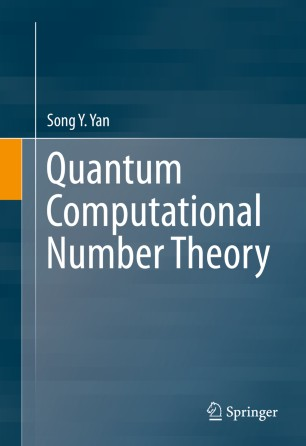# Quantum Computational Number TheoryBook

1. Front Matter
Pages i-ix
2. Song Y. Yan
Pages 1-31
3. Song Y. Yan
Pages 33-58
4. Song Y. Yan
Pages 59-119
5. Song Y. Yan
Pages 121-172
6. Song Y. Yan
Pages 173-228
7. Song Y. Yan
Pages 229-245
8. Back Matter
Pages 247-252

### Introduction

This book provides a comprehensive introduction to advanced topics in the computational and algorithmic aspects of number theory, focusing on applications in cryptography. Readers will learn to develop fast algorithms, including quantum algorithms, to solve various classic and modern number theoretic problems. Key problems include prime number generation, primality testing, integer factorization, discrete logarithms, elliptic curve arithmetic, conjecture and numerical verification.

The author discusses quantum algorithms for solving the Integer Factorization Problem (IFP), the Discrete Logarithm Problem (DLP), and the Elliptic Curve Discrete Logarithm Problem (ECDLP) and for attacking IFP, DLP and ECDLP based cryptographic systems. Chapters also cover various other quantum algorithms for Pell's equation, principal ideal, unit group, class group, Gauss sums, prime counting function, Riemann's hypothesis and the BSD conjecture.

Quantum Computational Number Theory is self-contained and intended to be used either as a graduate text in computing, communications and mathematics, or as a basic reference in the related fields. Number theorists, cryptographers and professionals working in quantum computing, cryptography and network security will find this book a valuable asset.

### Keywords

Quantum computational number theory Computational number theory Quantum computing Cryptography Computer algorithms Computational complexity theory Number-theoretic algorithms Integer factorization Discrete logarithm Elliptic curve arithmetic Public-key cryptography Design and analysis of algorithms Fast computation Digital signatures

#### Authors and affiliations

1. 1.Wuhan UniversityWuhanChina

### Bibliographic information

• Book Title Quantum Computational Number Theory
• Authors Song Y. Yan
• DOI https://doi.org/10.1007/978-3-319-25823-2
• Copyright Information Springer International Publishing Switzerland 2015
• Publisher Name Springer, Cham
• eBook Packages Computer Science Computer Science (R0)
• Hardcover ISBN 978-3-319-25821-8
• Softcover ISBN 978-3-319-79846-2
• eBook ISBN 978-3-319-25823-2
• Edition Number 1
• Number of Pages IX, 252
• Number of Illustrations 0 b/w illustrations, 40 illustrations in colour
• Topics
• Buy this book on publisher's site
Industry Sectors
Automotive
Biotechnology
IT & Software
Telecommunications
Consumer Packaged Goods
Aerospace
Engineering